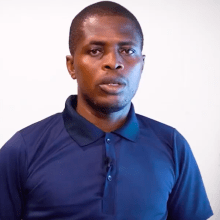SS3 Further Mathematics

## Matrices & determinants(2*2, 3*3) - continuation | Pure MathematicsInstructor John Ogbodo,   Certified Further Mathematics Instructor
##### Overview

Understanding the array as a linear transformation Finding determinants and apply to solve simultaneous equations in two or three unknowns

##### What you'll learn
• Basic definitions
• Matrices as linear transformations
• Determinants
• Solutions of 2 and 3 simultaneous equations.
##### Requirements
• No specific material is required.
##### Course content
More on Pure Mathematics
• Pure Mathematics 12 lessons